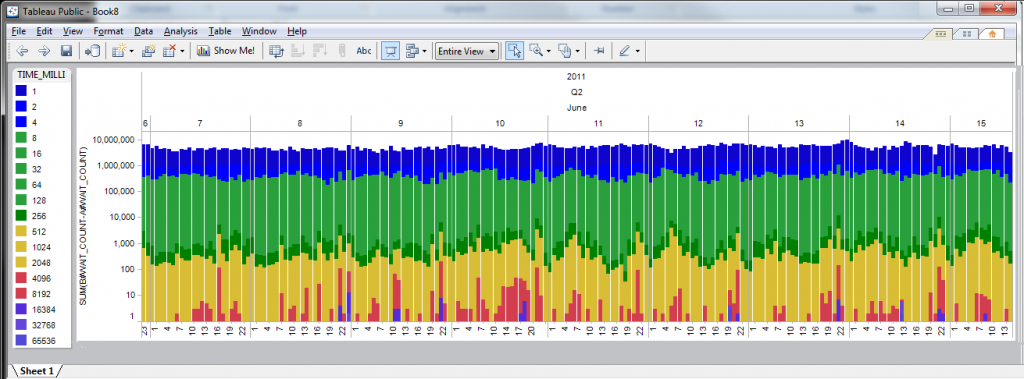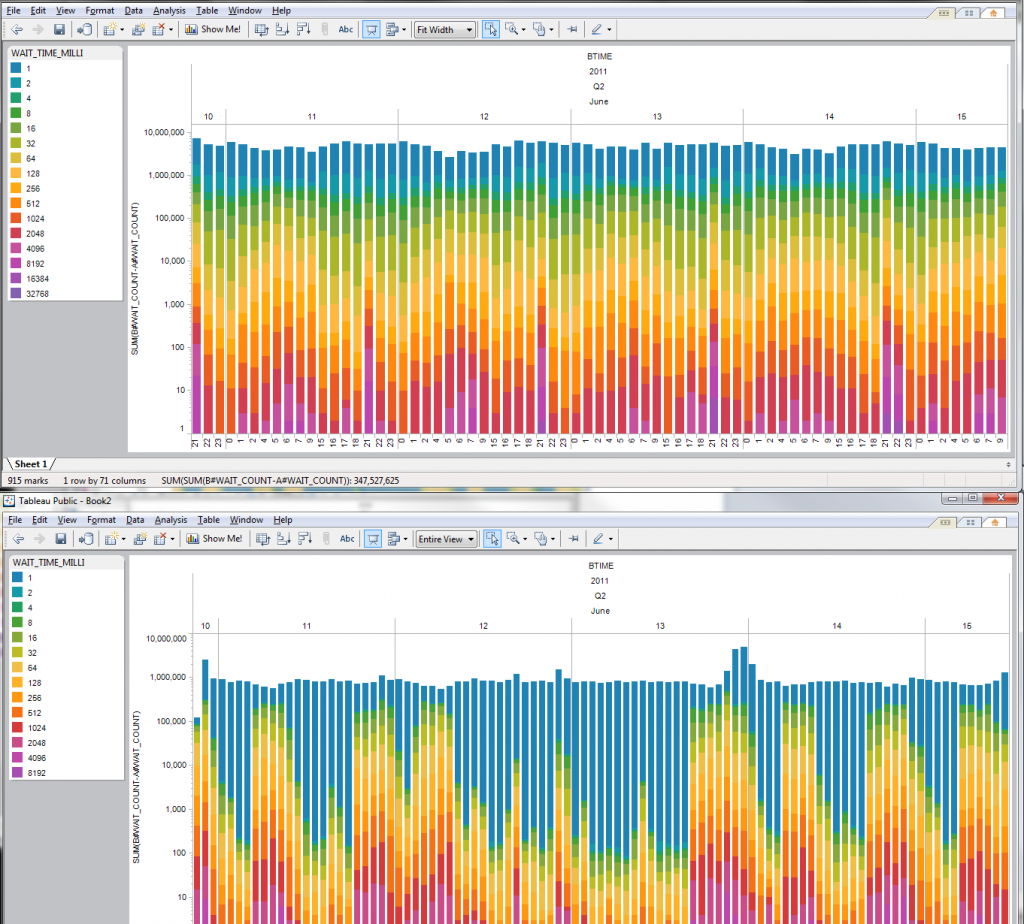## AWR mining – I/O wait histograms

June 21st, 2011

UPDATE: July 12, 2011  I just noticed (!) that the DBA_HIST_EVENT_HISTOGRAM is only on 11g, so for 10g, it requires collecting the histogram deltas manually off of V\$EVENT_HISTOGRAM, something like

```
set pagesize 150
col event format a25
col tm format a14
select event,
to_char(sysdate,'J')||to_char(round(sysdate-trunc(sysdate),5)) tm,
wait_time_milli,
wait_count
from v\$event_histogram
where event in ('log file parallel write' ,
order by event;```

and collecting this information every N seconds and taking the deltas. Also if you want the buckets in columns instead of rows:

```select
event,
to_char(sysdate,'J')||to_char(round(sysdate-trunc(sysdate),5)) tm,
sum (case when WAIT_TIME_MILLI=1 then WAIT_COUNT else 0 end) b1,
sum (case when WAIT_TIME_MILLI=2 then WAIT_COUNT else 0 end) b2,
sum (case when WAIT_TIME_MILLI=4 then WAIT_COUNT else 0 end) b3,
sum (case when WAIT_TIME_MILLI=8 then WAIT_COUNT else 0 end) b4,
sum (case when WAIT_TIME_MILLI=16 then WAIT_COUNT else 0 end) b5,
sum (case when WAIT_TIME_MILLI=32 then WAIT_COUNT else 0 end) b6,
sum (case when WAIT_TIME_MILLI=64 then WAIT_COUNT else 0 end) b7,
sum (case when WAIT_TIME_MILLI=128 then WAIT_COUNT else 0 end) b8,
sum (case when WAIT_TIME_MILLI=256 then WAIT_COUNT else 0 end) b9,
sum (case when WAIT_TIME_MILLI=512 then WAIT_COUNT else 0 end) b10,
sum (case when WAIT_TIME_MILLI=1024 then WAIT_COUNT else 0 end) b11,
sum (case when WAIT_TIME_MILLI=2048 then WAIT_COUNT else 0 end) b12,
sum (case when WAIT_TIME_MILLI=4096 then WAIT_COUNT else 0 end) b13,
sum (case when WAIT_TIME_MILLI=8192 then WAIT_COUNT else 0 end) b14,
sum (case when WAIT_TIME_MILLI=16384 then WAIT_COUNT else 0 end) b15,
sum (case when WAIT_TIME_MILLI=32768 then WAIT_COUNT else 0 end) b16,
sum (case when WAIT_TIME_MILLI=65536 then WAIT_COUNT else 0 end) b17,
sum (case when WAIT_TIME_MILLI=131072 then WAIT_COUNT else 0 end) b18,
sum (case when WAIT_TIME_MILLI=262144 then WAIT_COUNT else 0 end) b19,
sum (case when WAIT_TIME_MILLI=524288 then WAIT_COUNT else 0 end) b20,
sum (case when WAIT_TIME_MILLI=1048576 then WAIT_COUNT else 0 end) b21,
sum (case when WAIT_TIME_MILLI=2097152 then WAIT_COUNT else 0 end) b22,
sum (case when WAIT_TIME_MILLI=4194304 then WAIT_COUNT else 0 end) b23,
sum (case when WAIT_TIME_MILLI > 4194304 then WAIT_COUNT else 0 end) b24
from v\$event_histogram
where event='log file parallel write'
group by event,to_char(sysdate,'J')||to_char(round(sysdate-trunc(sysdate),5)) ;```

Exploring I/O histogram values in AWR. In the AWR repository I have several databases, all reading off the same LUNs, so below I try to  take the deltas between each snapshot and sum up all the waits for each bucket in the histogram

```set pagesize  50000
set feedback off
set echo off
SET markup HTML on
spool hist.html
with hist as  (
select
sn.snap_id,
sn.dbid,
to_char(trunc(cast(begin_interval_time as date ))+
(ROUND ((cast(begin_interval_time as  date) -
TRUNC (cast(begin_interval_time as date))) * 24) / 24),
'YYYY/MM/DD HH24:MI') btime,
h.event_name,
h.wait_time_milli,
h.wait_count
from dba_hist_event_histogram  h,
dba_hist_snapshot sn
where
h.instance_number = 1
and sn.instance_number = 1
and h.event_name like 'db file seq%'
and sn.snap_id=h.snap_id
and sn.dbid=h.dbid
)
select  a.btime,
a.wait_time_milli,
sum(b.wait_count - a.wait_count)
from hist a,
hist b
where a.dbid=b.dbid
and a.snap_id=b.snap_id-1
and a.wait_time_milli = b.wait_time_milli
group by a.btime, a.wait_time_milli
having  sum(b.wait_count - a.wait_count) > 0;
spool off
SET markup HTML off```

With the HTML output it’s easy to read into excel.
The data can be graphed in Excel using pivot tables or pivot charts, but if I simply save the data in an excel worksheet, then I can open it up in Tableau, which in some circumstances, can be easier to use than excelThe Y axis is in log scale. I don’t find that the data speaks to me immediately (other than there are some seriously slow I/Os) but it is easy to see the major outliers.

UPDATE:
One advantage of Tableau is the easy color coordination. Using the “cyclic” pallet, the colors come out pretty good for the I/O histograms (this would be time consuming on Excel)